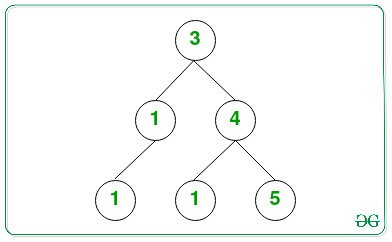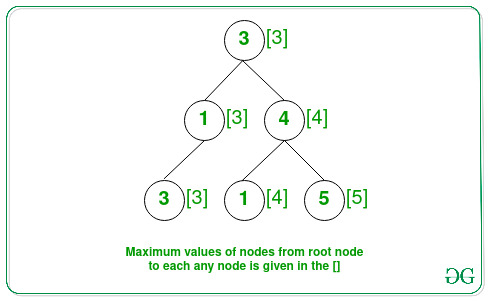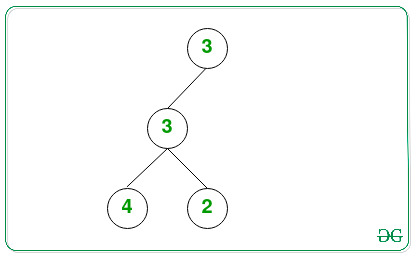# Count paths in a Binary Tree consisting of nodes in non-decreasing order

• Last Updated : 24 Sep, 2021

Given a Binary Tree consisting of N nodes, the task is to find the number of paths from the root to any node X, such that all the node values in that path are at most X.

Examples:

Input: Below is the given Tree:Output: 4
Explanation:The paths from the root to any node X that have value at most values of node X are:

• Node 3(root node): It always follows the given property.
• Node 4: The path starting from the root to node with value 4 has order (3 → 4), with the maximum value of a node being 4.
• Node 5: The path starting from the root to node with value 5 has order (3 → 4 → 5), with the maximum value of a node being 5.
• Node 3: The path starting from the root to node with value 3 has order (3 → 1 → 3), with the maximum value of a node being 3.

Therefore, the count of required paths is 4.

Input: Below is the given Tree:Output: 3

Approach – using DFS: The idea is to traverse the tree using a Depth First Search traversal while checking if the maximum value from root to any node X is equal to X or not.
Follow the steps below to solve the problem:

• Initialize a variable, say count as 0 to store the count of paths from the root to any node X having all the node values in that path is at most X.
• Traverse the tree recursively using depth-first-search and perform the following steps:
• Every recursive call for DFS Traversal, apart from the parent node, pass the maximum value of the node obtained so far in that path.
• Check if the current node value is greater or equal to the maximum value obtained so far, then increment the value of count by 1 and update the maximum value to the current node value.
• After completing the above steps, print the value of count as the result.

Below is the implementation of the above approach:

## C++

 `// C++ program for the above approach` `#include ``using` `namespace` `std;` `// Node structure of the binary tree``struct` `Node {``    ``int` `val;``    ``Node *left, *right;``};` `// Function for creating new node``struct` `Node* newNode(``int` `data)``{``    ``// Allocate memory for new node``    ``struct` `Node* temp = ``new` `Node();` `    ``// Assigning data value``    ``temp->val = data;``    ``temp->left = NULL;``    ``temp->right = NULL;` `    ``// Return the Node``    ``return` `temp;``}` `// Function to perform the DFS Traversal``// to find the number of paths having``// root to node X has value at most X``int` `countNodes(Node* root, ``int` `max)``{``    ``// If the root node is NULL``    ``if` `(!root)``        ``return` `0;` `    ``// Check if the current value is``    ``// greater than the maximum value``    ``// in path from root to current node``    ``if` `(root->val >= max)``        ``return` `1 + countNodes(root->left,``                              ``root->val)``               ``+ countNodes(root->right, root->val);` `    ``// Otherwise``    ``return` `countNodes(root->left,``                      ``max)``           ``+ countNodes(root->right,``                        ``max);``}` `// Driver Code``int` `main()``{``    ``// Given Binary Tree``    ``Node* root = NULL;``    ``root = newNode(3);``    ``root->left = newNode(1);``    ``root->right = newNode(4);``    ``root->left->left = newNode(3);``    ``root->right->left = newNode(1);``    ``root->right->right = newNode(5);` `    ``cout << countNodes(root, INT_MIN);` `    ``return` `0;``}`

## Java

 `// Java program for the above approach``import` `java.util.*;` `// Class containing left and``// right child of current``// node and key value``class` `Node {`` ` `    ``int` `data;``    ``Node left, right;`` ` `    ``public` `Node(``int` `item)``    ``{``        ``data = item;``        ``left = right = ``null``;``    ``}``}`` `  `class` `GFG {``    ` `    ``// Root of the Binary Tree``    ``Node root;`` ` `    ``public` `GFG()``    ``{``        ``root = ``null``;``    ``}``    ` `    ``// Function to perform the DFS Traversal``// to find the number of paths having``// root to node X has value at most X``static` `int` `countNodes(Node root, ``int` `max)``{``  ` `    ``// If the root node is NULL``    ``if` `(root == ``null``)``        ``return` `0``;` `    ``// Check if the current value is``    ``// greater than the maximum value``    ``// in path from root to current node``    ``if` `(root.data >= max)``        ``return` `1` `+ countNodes(root.left,``                              ``root.data)``               ``+ countNodes(root.right, root.data);` `    ``// Otherwise``    ``return` `countNodes(root.left,``                      ``max)``           ``+ countNodes(root.right,``                        ``max);``}` `  ``// Driver code``public` `static` `void` `main (String[] args)``{``  ` `      ``GFG tree = ``new` `GFG();``        ``tree.root = ``new` `Node(``3``);``        ``tree.root.left = ``new` `Node(``1``);``        ``tree.root.right = ``new` `Node(``4``);``        ``tree.root.left.left = ``new` `Node(``3``);``        ``tree.root.right.left = ``new` `Node(``1``);``        ``tree.root.right.right = ``new` `Node(``5``);``      ``System.out.println(countNodes(tree.root, Integer.MIN_VALUE));``    ``}``}` `// This code is contributed by offbeat`

## Python3

 `# Python3 program for the above approach` `# Node structure of the binary tree``class` `Node:``    ``def` `__init__(``self``, x):``        ``self``.val ``=` `x``        ``self``.left ``=` `None``        ``self``.right ``=` `None` `# Function to perform the DFS Traversal``# to find the number of paths having``# root to node X has value at most X``def` `countNodes(root, ``max``):``    ``# If the root node is NULL``    ``if` `(``not` `root):``        ``return` `0` `    ``# Check if the current value is``    ``# greater than the maximum value``    ``#in path from root to current node``    ``if` `(root.val >``=` `max``):``        ``return` `1` `+` `countNodes(root.left,root.val) ``+` `countNodes(root.right, root.val)` `    ``# Otherwise``    ``return` `countNodes(root.left, ``max``) ``+` `countNodes(root.right, ``max``)` `# Driver Code``if` `__name__ ``=``=` `'__main__'``:``  ` `    ``# Given Binary Tree``    ``root ``=` `Node(``3``)``    ``root.left ``=` `Node(``1``)``    ``root.right ``=` `Node(``4``)``    ``root.left.left ``=` `Node(``3``)``    ``root.right.left ``=` `Node(``1``)``    ``root.right.right ``=` `Node(``5``)` `    ``print``(countNodes(root, ``-``10``*``*``19``))` `# This code is contributed by mohit kumar 29.`

## C#

 `// C# program to count frequencies of array items``using` `System;` `// Class containing left and``// right child of current``// node and key value``class` `Node {`` ` `    ``public` `int` `data;``    ``public` `Node left, right;`` ` `    ``public` `Node(``int` `item)``    ``{``        ``data = item;``        ``left = right = ``null``;``    ``}``}`` ` `public` `class` `GFG``{``    ` `    ``// Root of the Binary Tree``    ``Node root;``    ``public` `GFG()``    ``{``        ``root = ``null``;``    ``}``    ` `// Function to perform the DFS Traversal``// to find the number of paths having``// root to node X has value at most X``static` `int` `countNodes(Node root, ``int` `max)``{``  ` `    ``// If the root node is NULL``    ``if` `(root == ``null``)``        ``return` `0;` `    ``// Check if the current value is``    ``// greater than the maximum value``    ``// in path from root to current node``    ``if` `(root.data >= max)``        ``return` `1 + countNodes(root.left,``                              ``root.data)``               ``+ countNodes(root.right, root.data);` `    ``// Otherwise``    ``return` `countNodes(root.left,``                      ``max)``           ``+ countNodes(root.right,``                        ``max);``}` `// Driver code``public` `static` `void` `Main(String []args)``{``        ``GFG tree = ``new` `GFG();``        ``tree.root = ``new` `Node(3);``        ``tree.root.left = ``new` `Node(1);``        ``tree.root.right = ``new` `Node(4);``        ``tree.root.left.left = ``new` `Node(3);``        ``tree.root.right.left = ``new` `Node(1);``        ``tree.root.right.right = ``new` `Node(5);``        ``Console.WriteLine(countNodes(tree.root, Int32.MinValue));``    ``}``}` `// This code is contributed by jana_sayantan.`

## Javascript

 ``
Output:
`4`

Time Complexity: O(N)
Auxiliary Space: O(N)

Approach using BFS: The idea is to traverse the tree using breadth-first search while checking if the maximum value from root to X is equal to X. Follow the steps below to solve the problem:

• Initialize a variable, say count as 0 to store the count of paths from the root to any node X having all the node values in that path is at most X and a queue Q of pairs to perform the BFS Traversal.
• Push the root node with INT_MIN as the maximum value in the queue.
• Now, until Q is non-empty perform the following:
• Pop the front node from the queue.
• If the front node value is at least the current maximum value obtained so far, then increment the value of count by 1.
• Update the maximum value that occurred so far with the current node value.
• If the left and right nodes exist for the current popped node then push it into the queue Q with the updated maximum value in the above step.
• After completing the above steps, print the value of count as the result.

Below is the implementation of the above approach:

## C++

 `// C++ program for the above approach` `#include ``using` `namespace` `std;` `// Node of the binary tree``struct` `Node {``    ``int` `val;``    ``Node *left, *right;``};` `// Function for creating new node``struct` `Node* newNode(``int` `data)``{``    ``// Allocate memory for new node``    ``struct` `Node* temp = ``new` `Node();``    ``temp->val = data;``    ``temp->left = NULL;``    ``temp->right = NULL;` `    ``// Return the created node``    ``return` `temp;``}` `// Function to perform the DFS Traversal``// to find the number of paths having``// root to node X has value at most X``int` `countNodes(Node* root)``{``    ``// Initialize queue``    ``queue > q;``    ``int` `m = INT_MIN;` `    ``// Push root in queue with the``    ``// maximum value m``    ``q.push({ root, m });` `    ``// Stores the count of good nodes``    ``int` `count = 0;` `    ``// Traverse all nodes``    ``while` `(!q.empty()) {` `        ``// Store the front node of``        ``// the queue``        ``auto` `temp = q.front();``        ``q.pop();``        ``Node* node = temp.first;``        ``int` `num = temp.second;` `        ``// Check is current node is``        ``// greater than the maximum``        ``// value in path from root to``        ``// the current node``        ``if` `(node->val >= num)``            ``count++;` `        ``// Update the maximum value m``        ``m = max(node->val, num);` `        ``// If left child is not null,``        ``// push it to queue with the``        ``// maximum value m``        ``if` `(node->left)``            ``q.push({ node->left, m });` `        ``// If right child is not null,``        ``// push it to queue with the``        ``// maximum value m``        ``if` `(node->right)``            ``q.push({ node->right, m });``    ``}` `    ``// Returns the answer``    ``return` `count;``}` `// Driver Code``int` `main()``{``    ``// Construct a Binary Tree``    ``Node* root = NULL;``    ``root = newNode(3);``    ``root->left = newNode(1);``    ``root->right = newNode(4);``    ``root->left->left = newNode(3);``    ``root->right->left = newNode(1);``    ``root->right->right = newNode(5);` `    ``cout << countNodes(root);` `    ``return` `0;``}`

## Java

 `// Java program for the above approach``import` `java.util.*;` `// Node of the binary tree``class` `Node {``  ` `    ``int` `val;``    ``Node left, right;``  ` `    ``public` `Node(``int` `data)``    ``{``        ``val = data;``        ``left = right = ``null``;``    ``}``}` `// User defined Pair class``class` `Pair {``    ``Node x;``    ``int` `y;``  ` `    ``// Constructor``    ``public` `Pair(Node x, ``int` `y)``    ``{``        ``this``.x = x;``        ``this``.y = y;``    ``}``}``    ` `public` `class` `Main``{``    ``// Function for creating new node``    ``static` `Node newNode(``int` `data)``    ``{``        ``// Allocate memory for new node``        ``Node temp = ``new` `Node(data);``      ` `        ``// Return the created node``        ``return` `temp;``    ``}``      ` `    ``// Function to perform the DFS Traversal``    ``// to find the number of paths having``    ``// root to node X has value at most X``    ``static` `int` `countNodes(Node root)``    ``{``        ``// Initialize queue``        ``Vector q = ``new` `Vector();``        ``int` `m = Integer.MIN_VALUE;``      ` `        ``// Push root in queue with the``        ``// maximum value m``        ``q.add(``new` `Pair(root, m));``      ` `        ``// Stores the count of good nodes``        ``int` `count = ``0``;``      ` `        ``// Traverse all nodes``        ``while` `(q.size() > ``0``) {``      ` `            ``// Store the front node of``            ``// the queue``            ``Pair temp = q.get(``0``);``            ``q.remove(``0``);``            ``Node node = temp.x;``            ``int` `num = temp.y;``      ` `            ``// Check is current node is``            ``// greater than the maximum``            ``// value in path from root to``            ``// the current node``            ``if` `(node.val >= num)``                ``count++;``      ` `            ``// Update the maximum value m``            ``m = Math.max(node.val, num);``      ` `            ``// If left child is not null,``            ``// push it to queue with the``            ``// maximum value m``            ``if` `(node.left != ``null``)``                ``q.add(``new` `Pair(node.left, m));``      ` `            ``// If right child is not null,``            ``// push it to queue with the``            ``// maximum value m``            ``if` `(node.right != ``null``)``                ``q.add(``new` `Pair(node.right, m));``        ``}``      ` `        ``// Returns the answer``        ``return` `count;``    ``}` `    ``public` `static` `void` `main(String[] args) {``        ``// Construct a Binary Tree``        ``Node root = ``null``;``        ``root = newNode(``3``);``        ``root.left = newNode(``1``);``        ``root.right = newNode(``4``);``        ``root.left.left = newNode(``3``);``        ``root.right.left = newNode(``1``);``        ``root.right.right = newNode(``5``);``      ` `        ``System.out.println(countNodes(root));``    ``}``}` `// This code is contributed by mukesh07.`

## Python3

 `# Python3 program for the above approach``import` `sys` `# Node of the binary tree``class` `Node:``    ``def` `__init__(``self``, data):``        ``self``.val ``=` `data``        ``self``.left ``=` `None``        ``self``.right ``=` `None` `# Function for creating new node``def` `newNode(data):``    ``# Allocate memory for new node``    ``temp ``=` `Node(data)` `    ``# Return the created node``    ``return` `temp` `# Function to perform the DFS Traversal``# to find the number of paths having``# root to node X has value at most X``def` `countNodes(root):``    ``# Initialize queue``    ``q ``=` `[]``    ``m ``=` `-``sys.maxsize` `    ``# Push root in queue with the``    ``# maximum value m``    ``q.append([ root, m ])` `    ``# Stores the count of good nodes``    ``count ``=` `0` `    ``# Traverse all nodes``    ``while` `(``len``(q) > ``0``):``        ``# Store the front node of``        ``# the queue``        ``temp ``=` `q[``0``]``        ``q.pop(``0``)``        ``node ``=` `temp[``0``]``        ``num ``=` `temp[``1``]` `        ``# Check is current node is``        ``# greater than the maximum``        ``# value in path from root to``        ``# the current node``        ``if` `(node.val >``=` `num):``            ``count``+``=``1` `        ``# Update the maximum value m``        ``m ``=` `max``(node.val, num)` `        ``# If left child is not null,``        ``# push it to queue with the``        ``# maximum value m``        ``if` `(node.left !``=` `None``):``            ``q.append([ node.left, m ])` `        ``# If right child is not null,``        ``# push it to queue with the``        ``# maximum value m``        ``if` `(node.right !``=` `None``):``            ``q.append([ node.right, m ])` `    ``# Returns the answer``    ``return` `count` `# Construct a Binary Tree``root ``=` `None``root ``=` `newNode(``3``)``root.left ``=` `newNode(``1``)``root.right ``=` `newNode(``4``)``root.left.left ``=` `newNode(``3``)``root.right.left ``=` `newNode(``1``)``root.right.right ``=` `newNode(``5``)` `print``(countNodes(root))` `# This code is contributed by rameshtravel07.`

## C#

 `// C# program for the above approach``using` `System;``using` `System.Collections;``class` `GFG {``    ` `    ``// Node of the binary tree``    ``class` `Node {``       ` `        ``public` `int` `val;``        ``public` `Node left, right;``       ` `        ``public` `Node(``int` `data)``        ``{``            ``val = data;``            ``left = right = ``null``;``        ``}``    ``}``    ` `    ``// Function for creating new node``    ``static` `Node newNode(``int` `data)``    ``{``        ``// Allocate memory for new node``        ``Node temp = ``new` `Node(data);``     ` `        ``// Return the created node``        ``return` `temp;``    ``}``     ` `    ``// Function to perform the DFS Traversal``    ``// to find the number of paths having``    ``// root to node X has value at most X``    ``static` `int` `countNodes(Node root)``    ``{``        ``// Initialize queue``        ``Queue q = ``new` `Queue();``        ``int` `m = Int32.MinValue;``     ` `        ``// Push root in queue with the``        ``// maximum value m``        ``q.Enqueue(``new` `Tuple(root, m));``     ` `        ``// Stores the count of good nodes``        ``int` `count = 0;``     ` `        ``// Traverse all nodes``        ``while` `(q.Count > 0) {``     ` `            ``// Store the front node of``            ``// the queue``            ``Tuple temp = (Tuple)q.Peek();``            ``q.Dequeue();``            ``Node node = temp.Item1;``            ``int` `num = temp.Item2;``     ` `            ``// Check is current node is``            ``// greater than the maximum``            ``// value in path from root to``            ``// the current node``            ``if` `(node.val >= num)``                ``count++;``     ` `            ``// Update the maximum value m``            ``m = Math.Max(node.val, num);``     ` `            ``// If left child is not null,``            ``// push it to queue with the``            ``// maximum value m``            ``if` `(node.left != ``null``)``                ``q.Enqueue(``new` `Tuple(node.left, m));``     ` `            ``// If right child is not null,``            ``// push it to queue with the``            ``// maximum value m``            ``if` `(node.right != ``null``)``                ``q.Enqueue(``new` `Tuple(node.right, m));``        ``}``     ` `        ``// Returns the answer``        ``return` `count;``    ``}``    ` `  ``// Driver code``  ``static` `void` `Main()``  ``{``    ` `    ``// Construct a Binary Tree``    ``Node root = ``null``;``    ``root = newNode(3);``    ``root.left = newNode(1);``    ``root.right = newNode(4);``    ``root.left.left = newNode(3);``    ``root.right.left = newNode(1);``    ``root.right.right = newNode(5);`` ` `    ``Console.Write(countNodes(root));``  ``}``}` `// This code is contributed by decode2207.`

## Javascript

 ``
Output:
`4`

Time Complexity: O(N)
Auxiliary Space: O(N)

My Personal Notes arrow_drop_up